# Motion of a charged particle in magnetic field

We have read about the interaction of electric field and magnetic field and the motion of charged particles in the presence of both the electric and magnetic fields and also have derived the relation of the force acting on the charged particle, in this case, given by Lorentz force. We have also read about the magnetic force on a current-carrying conducting rod when placed in a magnetic field. But what happens when a charged particle moves in the presence of a magnetic field? How do we define the trajectory of such a particle? In this section, we will learn about this in detail.

A force acting on a particle is said to perform work when there is a component of the force in the direction of motion of the particle. In the case under consideration where we have a charged particle carrying a charge q moving in a uniform magnetic field of magnitude B, the magnetic force acts perpendicular to the velocity of the particle. Here we say that no work is done by the magnetic force on the particle and hence, no change in the velocity of the particle can be seen. Mathematically, when the velocity of the particle v is perpendicular to the direction of the magnetic field, we can write,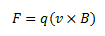Here, the magnetic force is directed towards the center of circular motion undergone by the object and acts as a centripetal force. Thus, if v and B are perpendicular to each other, the particle describes a circle. In other cases, when a component of velocity is present along the direction of the magnetic field B, then its magnitude remains unchanged throughout the motion, as no effect of a magnetic field is felt upon it. Also, the motion due to the perpendicular component of the velocity is circular in nature, as discussed above. The resulting motion due to the two components is a helical motion, as shown in the image below.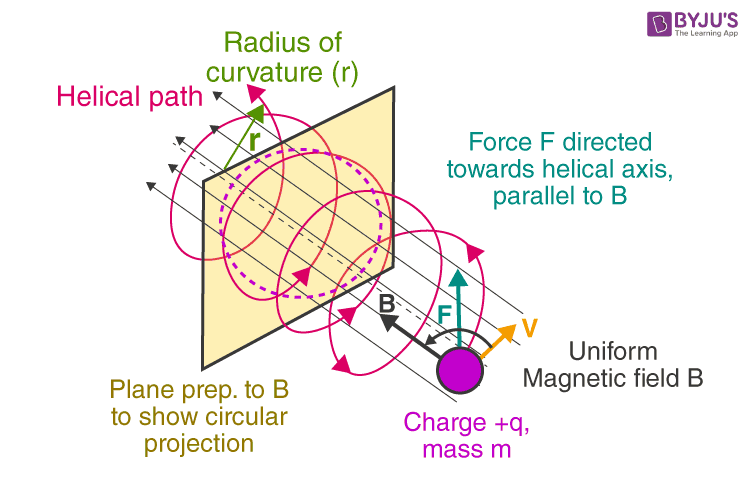As the radius of the circular path of the particle is r, the centripetal force acting perpendicular to it towards the center can be given as,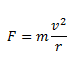Also, the magnetic force acts perpendicular to both the velocity and the magnetic field and the magnitude can be given as,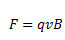Equating the two, we get,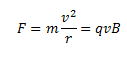Or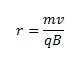Here, r gives the radius of the circle described by the particle. Also, if we write the angular frequency of the particle as ω, then we can write,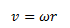So,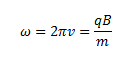Here, v is the frequency of rotation of the particle. The time for one revolution can be given as,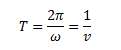The distance moved by the particle along the direction of the magnetic field in one rotation is given by its pitch.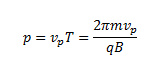Where vp is the velocity parallel to the magnetic field.

To know more about the motion of charged particles in a magnetic field, click on the video below.Test your Knowledge on Motion Charged Particle Magnetic Field